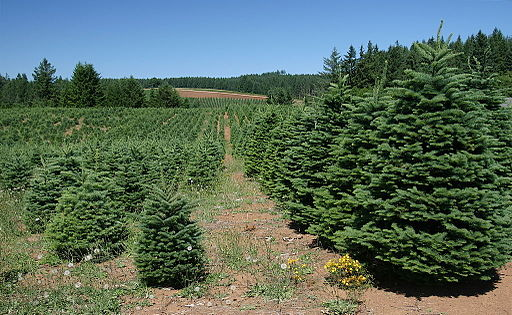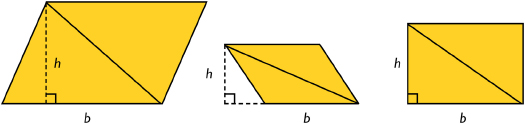# Analyzing The Formula of A Triangle

Student View (Opens in new window)# Lesson Overview

Students find the area of a triangle by putting together a triangle and a copy of the triangle to form a parallelogram with the same base and height as the triangle. Students also create several examples of triangles and look for relationships among the base, height, and area measures. These activities lead students to develop and understand a formula for the area of a triangle.

# Key Concepts

To find the area of a triangle, you must know the length of a base and the corresponding height. The base of a triangle can be any of the three sides. The height is the perpendicular distance from the vertex opposite the base to the line containing the base. The height can be found inside or outside the triangle, or it can be the length of one of the sides.

You can put together a triangle and a copy of the triangle to form a parallelogram with the same base and height as the triangle. The area of the original triangle is half of the area of the parallelogram. Because the area formula for a parallelogram is A = bh, the area formula for a triangle is A = $\frac{1}{2}$bh.# Goals and Learning Objectives

• Develop and explore the formula for the area of a triangle.